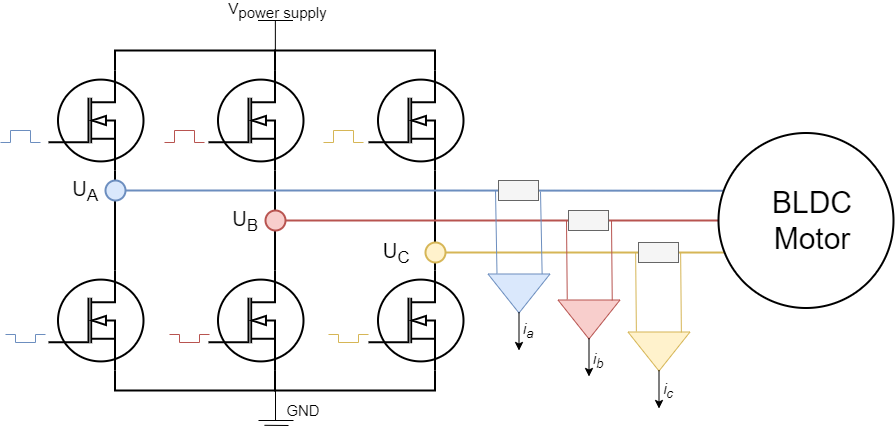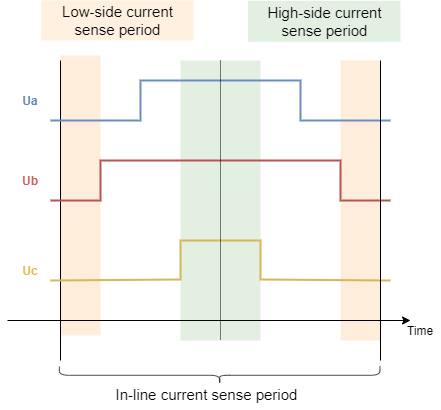# In-line current sensing

Inline current sensing technique is the simplest one to use and the most precise one. The shunt resistors are placed in-line with motor phases and the current measured on these shunt resistors will be motor phase current regardless of the state of the PWM duty cycle. This implementation is therefore very suitable for Arduino devices due to the fact that adc can be sampled at any time to read the current and the adc acquisition duration is as important as for the other current sensing approaches. The downside of this approach is the hardware, this current sensing architecture requirers high-precision bidirectional amplifiers with much better PWM rejection than the regular low-side or high-side amplifiers.## Step 1. Hardware configuration

``````// InlineCurrentSensor constructor
//  - shunt_resistor  - shunt resistor value
//  - gain  - current-sense op-amp gain
//  - phA   - A phase adc pin
//  - phB   - B phase adc pin
//  - phC   - C phase adc pin (optional)
InlineCurrentSense current_sense  = InlineCurrentSense(0.01, 20, A0, A1, A2);
``````

To instantiate the inline current sensor using the SimpleFOClibrary just create an instance of the class `InlineCurrentSense`. This class takes as a parameter shunt resistance value `shunt_resistor`, amplification gain `gain` and two or three ADC channel pins depending on the available measuring hardware that you might have. It is important to specify right adc channels for right driver/motor phase. So if your pin `A0` measures the phase current `A` and pin `A1` measures the phase current `B` make sure to put provide them to the constructor in that order.

Field Oriented Control algorithm can run with both 2 or 3 phase current measurements.

The constructor of the `InlineCurrentSense` class only allows you to specify one shunt resistor value and one amplification gain. If your hardware configuration has different shunt/amp values for different phases you can specify them by changing the `gain_x` attribute:

``````// default values of per phase gains
current_sense.gain_a = 1.0 / shunt_resistor / gain;
current_sense.gain_b = 1.0 / shunt_resistor / gain;
current_sense.gain_c = 1.0 / shunt_resistor / gain;
``````

For example Arduino SimpleFOCShield v2 has the phase B of the current sensing inverted. So in its case you can specify:

``````// inverse current sensing gain on phase b
current_sense.gain_b *= -1;
``````

Once the current sense has been created it can be initialised. This `init()` function configures the ADC hardware for reading and finds the zero offsets of the ADC for each channel.

``````// init current sense
current_sense.init();
``````

Once when your current sense has been intialised and calibrated you can start measuring the currents!

## Using the current sense with FOC algorithm

To use the `InlineCurrentSense` with the FOC algorithm all you need to do is to add it to link it with the `BLDCMotor` you wish to use it with:

``````// link motor and current sense
``````

The `BLDCMotor` class in the `initFOC()` function where it aligns all the sensors, will align the `InlineCurrentSense` with the `BLDCDriver` that is linked to the motor.

``````// prepare sensors for FOC
motor.initFOC();
``````

Function `initFOC()` will call two current sense class functions that are important:

• `current_sense.driverSync(...)`
• `current_sense.driverAlign(...)`

### Driver synchronisation `driverSync(...)`Since the Inline current sensing technique does not require triggering of particular events for ADC acquisition the `driverSync()` function does nothing really. This function will be very ipmportant for low-side and high-side current sensing as shown on the figure above.

### Alignment with the motor phases `driverAlign(...)`

The alignment is done by calling the function:

``````current_sense.driverAlign(&driver, voltage_sensor_align);
``````

This function applies using the driver instance applies the voltage to each of the phases and checks if the measured currents correspond to the directions of the applied voltages. This alignment procedure is able to correct for:

• Incorrect order of adc pins
• Incorrect gain sign

If monitoring is enabled for the motor during the `initFOC` the monitor will display the alignment status:

• `0` - fail
• `1` - success and nothing changed
• `2` - success but pins reconfigured
• `3` - success but gains inverted
• `4` - success but pins reconfigured and gains inverted

If you are sure in your configuration and if you wish to skip the alignment procedure you can specify set the `skip_align` flag before calling `motor.initFOC()`:

``````// skip alignment procedure
current_sense.skip_align = true;
``````

For example Arduino SimpleFOCShield v2, you would have a code similar to this:

``````// invert phase b gain
current_sense.gain_b *=-1;
// skip alignment
current_sense.skip_align = true;
...
// align all sensors
motor.initFOC();
``````

## Standalone current sense

To use your inline current sensor as a stand-alone sensor after you have configured the hardware and calibrated it you can read the phase currents by calling:

``````PhaseCurrent_s  current = current_sense.getPhaseCurrents();
``````

This function returns the `PhaseCurrent_s` structure that which has three variables `a`, `b` and `c`. So you can print them out for example;

``````Serial.println(current.a);
Serial.println(current.b);
Serial.println(current.c); // 0 if only two currents mode
``````

If you are using only two phase current measurements in your `InlineCurrentSense`, it will return the third current `current.c` equal to 0.

Sometimes the phase currents are hard to interpret, therefore this current sense class enables you to read the transformed current vector magnitude. The absolute DC current the motor is drawing.

``````float current_mag = current_sense.getDCCurrent();
``````

Futhermore if you have an access to the position sensor of the motor connected to the driver you can get signed value of the DC current the motor is drawing by providing it to the `getDCCurrent` method.

``````float current = current_sense.getDCCurrent(motor_electrical_angle);
``````

Finally if you have the access to the motor position sensor you current sense class will be able to tell you the FOC currents D and Q that your motor is drawing.

``````DQCurrent_s current = current_sense.getFOCCurrents(motor_electrical_angle);
``````

This function returns the `DQCurrent_s` structure which has two variables, `d` and `q`. You can print them out for example:

``````Serial.println(current.d);
Serial.println(current.q);
``````

### Example code

Here is a simple example of a inline current sense as a stand-alone sensor using the SimpleFOClibrary and SimpleFOCShield v2.

``````#include <SimpleFOC.h>

// current sensor
// shunt resistor value
// gain value
// pins phase A,B
InlineCurrentSense current_sense = InlineCurrentSense(0.01, 50.0, A0, A2);

void setup() {
// initialise the current sensing
current_sense.init();

// for SimpleFOCShield v2.01/v2.0.2
current_sense.gain_b *= -1;

Serial.begin(115200);
}

void loop() {

PhaseCurrent_s currents = current_sense.getPhaseCurrents();
float current_magnitude = current_sense.getCurrent();

Serial.print(currents.a*1000); // milli Amps
Serial.print("\t");
Serial.print(currents.b*1000); // milli Amps
Serial.print("\t");
Serial.print(currents.c*1000); // milli Amps
Serial.print("\t");
Serial.println(current_magnitude*1000); // milli Amps
}

``````